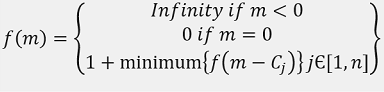Q:

# Minimum Number of coins to make the change

Given a value P, you have to make change for P cents, given that you have infinite supply of each of C { C1, C2, ... ,Cn} valued coins. Find the minimum number of coins to make the change. Return -1 if the change is not possible with the coins provided.

```    Input:
Amount P=13

Coin values are:
1, 4, 5

Output:
3
```

Explanation with example

Let's solve the above example. Total amount is 13 and we have coins with values 1, 4 and 5.

Since, we are to find minimum number of coins and we have infinite number of coin for any denomination, it's apparent that we would go for greedy. We should pick the coin with maximum denomination and keep trying with that until the remaining amount of the change is less the denomination of the coin. Then on course we keep choosing the next best one. So, the algorithm would be like.

```    1) Let, count=0 to count minimum number of coin used
2) Pick up coin with maximum denomination say, value x
3) while amount≥x
amount=amount-x
count=count+1

4) if amount=0
Go to Step 7
5) Pick up the next best denomination of coin and assign it to x
6) Go to Step 2
7) End. count is the minimum number of coin used.
```

Let's see whether the above greedy algorithm leads to the desired result or not.

```    So, we would pick up 5 first from {1, 4, 5}
We would pick 5 two times
Now amount=3, count=2
Next best coin is 4 but 4 > amount
Next best coin is 1
We would pick 1 three times
Amount=0 and count=5
So, minimum number of coins needed for the change is 5.

But is it really minimum?
If we choose one coin with denomination 5 and two coins with denomination 4,
it would make the change and coins needed here is (2+1) = 3
So, greedy does not work as the local optimum choices doesn't make
global optimum choice. Hence, we need dynamic programming.```

We have amount M and n number of coins, {C1, C2, ..., Cn}

Now,

We have two choice for any Cj,

1. Use Cj and recur for amount M-Cj
2. Don't use Cj and recur for amount M with other coins

(m)= minimum number of coins needed to change amount mWe can formulate the above recursion using DP.

```    // (for any amount 0 to M, minimum number of coins needed is initially infinity)
1) Initialize DP[M+1] with INT_MAX.
2) DP=0 //base case of above recursion
3)  for  i=1 to M //iterate amounts
for any coin C_j
If i>=C_j  && DP[i-C_j]≠INT_MAX && DP[i-C_j]+1<DP[i])
DP[i]=DP[i-C_j]+1; //update value
End for
End for

The result is DP[M]
```

C++ implementation:

``````#include <bits/stdc++.h>
using namespace std;

int coin_change(vector<int> a, int m, int n)
{

//////////////////recursive implementation//////////////
// if(m<0)
// return;
// if(m==0){
//     if(count<min_count)
//     min_count=count;
//     return;
// }

// for(int i=0;i<n;i++){
//     coin_change(a,i,m-a[i],n,count+1);
//     coin_change(a,i+1,m-a[i],n,count+1);
// }

///////////DP implementation///////////////
//base value
int DP[m + 1];
//initialize
for (int i = 1; i <= m; i++)
DP[i] = INT_MAX;
DP = 0;
for (int i = 1; i <= m; i++) {
for (int j = 0; j < n; j++) {
// if amount > coin value
if (i >= a[j] && DP[i - a[j]] != INT_MAX && DP[i - a[j]] + 1 < DP[i])
// if updation possible update for minimum value
DP[i] = DP[i - a[j]] + 1;
}
}

return DP[m];
}
int main()
{
int n, item, m;

cout << "Enter amount to be changes:\n";
cin >> m;
cout << "Enter number of coins:\n";
cin >> n;
cout << "Enter coin values\n";
vector<int> a;
for (int j = 0; j < n; j++) {
scanf("%d", &item);
a.push_back(item);
}
int ans = coin_change(a, m, n);
if (ans == INT_MAX)
cout << "Coin change not possible" << endl;
else
cout << "Minimum number of coins needed: " << ans << endl;

return 0;
}
``````

Output

```RUN 1:
Enter amount to be changes:
13
Enter number of coins:
3
Enter coin values
1 4 5
Minimum number of coins needed: 3

RUN 2:
Enter amount to be changes:
11
Enter number of coins:
2
Enter coin values
4 5
Coin change not possible```